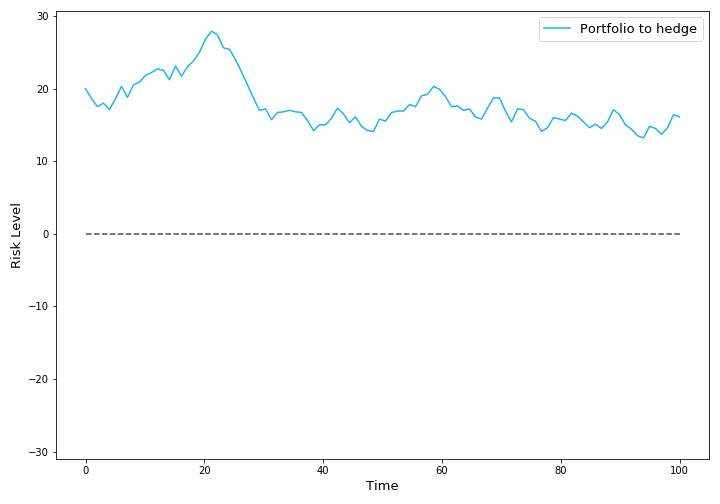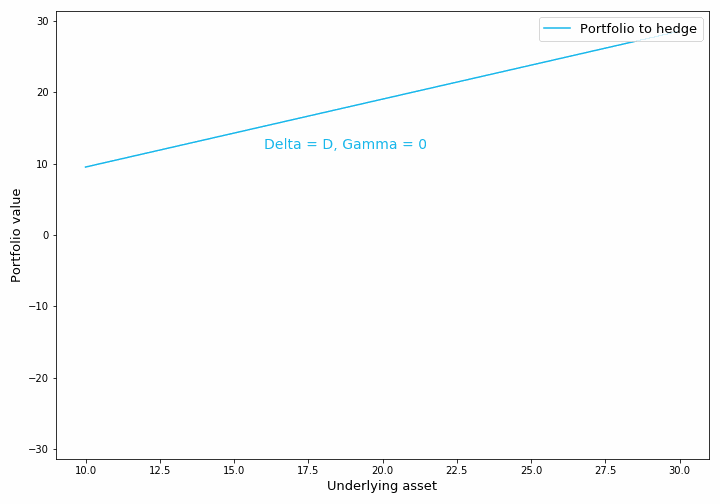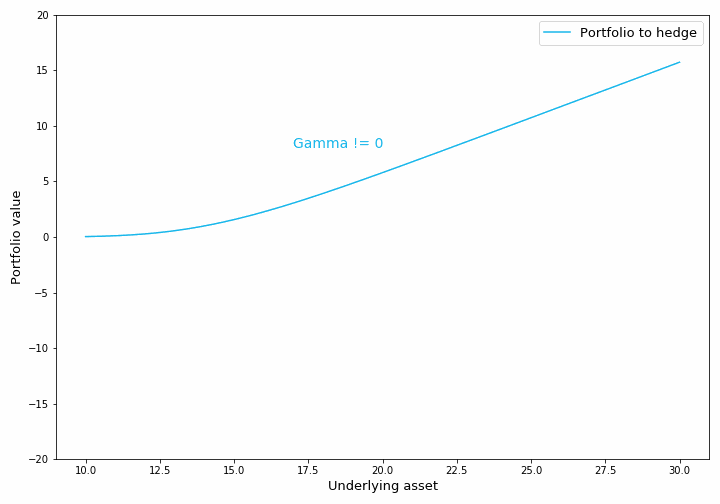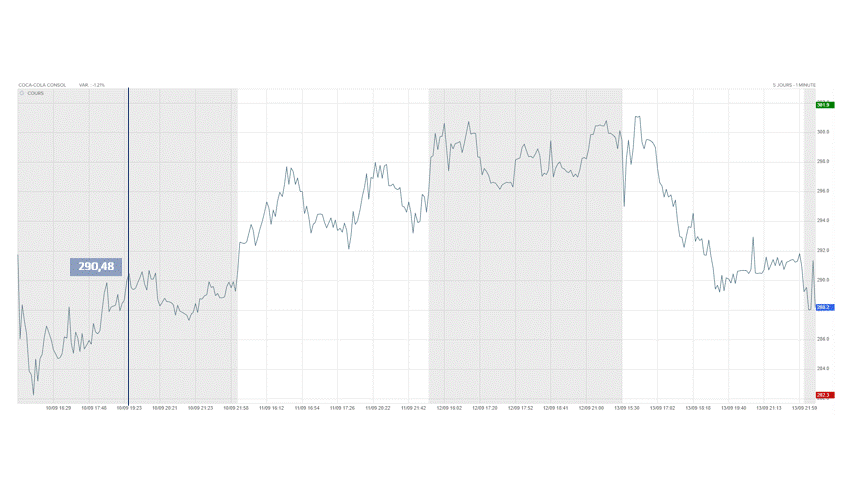Search

# Risk management and Gamma-Hedging

Updated: Jun 28, 2021

Risk management in derivatives trading is as essential as it is complex.

The price at which a derivative product is bought or sold on an exchange depends on several parameters. These parameters can be divided into two categories:

• The characteristics of the derivative. E.g.: its rate if it is a bond, its strike if it is an option, its maturity etc.

• The market variables used to compute its price. E.g.: the price of one or more underlying assets, interest rates, etc.

## Hedging risksTo hedge the risks of derivatives, the trading book must be made as insensitive as possible to market movements. This is why the trader builds another portfolio, called a hedging portfolio, to obtain a Global Trading Book (sum of both portfolios) that is almost risk-free.

To construct an effective hedging portfolio, it is necessary to compute the sensitivities of the derivative product prices with respect to the different market variables; in other words, to compute the derivatives of the price function with respect to its parameters.

Hedging a book against the variations of a variable is equivalent to cancelling out the associated derivative.

Some derivatives that are commonly used in finance include:

• Delta: the first derivative of the price relative to an underlying asset

• Gamma: the second derivative of the price in relation to an underlying asset.

• Vega: the first derivative of the price relative to the volatility of an underlying asset.

• Theta: the first derivative of price versus time.

## Delta and Gamma hedging

One of the most obvious reasons for the price of a derivative to change is that the values of its underlying assets have changed themselves. To hedge a portfolio against the price variations of an underlying asset, the first step is to Delta hedge the portfolio, which means to cancel out its Delta.

To explain Delta hedging, we will take simple examples with only one derivative product to hedge, based on a single underlying asset.

We observe that the current value of the asset is 15€ so we will compute our Delta with this value.Example: In this first example, the portfolio contains one future, which is a derivative product with a linear price w.r.t. the value of its underlying. So, the derivative, Delta, is a constant D.

If the hedging portfolio is built to have a Delta of -D, then the global trading book will have a Delta of 0.

In this case, regardless of the changes in the underlying price, the global book will have the same value.

All risk has been eliminated in this simple example because the price is linear in the underlying asset. But what happens when this is not the case?

### What is Gamma-Hedging?

When the Gamma of the portfolio to hedge is not zero (this is the case for many derivative products), Delta hedging the portfolio is not enough.

Let's get back to our previous example and replace the future (with a linear price) by an option (with a non-linear one).

In this example again, we compute the Delta when the asset value is 15€ and build the hedging portfolio accordingly.In the graph above, the hedging portfolio covers risks only if the underlying does not move away too much from its initial value, 15€.

If the asset value is at 23€ (or at 10€), the trading book is not covered anymore, the new value of Delta must be recomputed, and the hedging portfolio modified accordingly. This operation is called rebalancing the hedging portfolio.

In other words, due to the portfolio Gamma, the Delta hedging operation must be repeated regularly: this is Gamma hedging.

Now, you know what you have to do, but you don't know how to do it yet!

### Difficulties

Difficulties in setting up and executing a Gamma hedging strategy are:

1. How to calculate the Delta and the Gamma? What is the composition of the hedging portfolio?

2. When to rebalance the portfolio to hedge enough without paying too many transaction fees?

## What are the different strategies?

• Theoretical strategy: continuous hedging

In theory, the hedging could be perfect (without any risk) if we could Gamma hedge the portfolio continuously. We could approach this theoretical strategy by rebalancing very frequently, every second or every minute, but this is not effective due to transaction costs. This strategy is therefore not replicable in practice.

Traders has their own expertise and experience. Many therefore use their own strategy, based on their analysis of the market.

• Threshold strategies

To avoid rebalancing the hedging portfolio when it is not necessary, the trader may set thresholds on some indicators (the underlying spot, the Delta of the global portfolio etc.) and rebalance only when the threshold is reached.Coca-Cola spot / Spot Threshold

In the example above, we show the rebalancing moments (depicted by vertical lines) of a rebalancing strategy set at +/- 2% of spot variation. For this type of strategy, the difficulty lies in choosing the right threshold level.

• TEMPO Gamma-Hedging

Tempo is the solution designed and developed by Kesitys for intraday hedging.

This is a solution that analyses real-time derivatives portfolio and market data and provides the trader with the optimal moments to rebalance his hedging portfolio. These moments are computed to minimize transaction costs without increasing the level of risk compared to a benchmark solution chosen by the trader.

To find out more about Tempo, how it works and its performance, it's here!

### Close strategies

Sometimes, intraday operations are not possible, and transactions are only carried out at the time of market closure. In some cases, this is because the trader has no intraday market access, in others, this is a business choice because liquidity is very high at the close.

• Close-to-Close or Delta-Flat strategy

This strategy is the simplest but probably the most widely used. It consists in rebalancing the hedging portfolio at each market close and completely cancelling out the Delta.Coca-Cola spot / Close-to-Close Hedging
• COMBO Gamma-Hedging

Combo is a solution designed and developed by Kesitys. It is an optimised solution that changes the composition of the Close-to-Close hedging portfolio and the frequency of rebalancing to minimize transaction costs, while controlling the level of risk.

To find out more about Combo, how it works and its performance, it's here!